Home > A2C > Chapter 7 > Lesson 7.2.1 > Problem7-98

7-98.
1. Consider the questions below. Homework Help ✎

1. What can you multiply 8 by to get 1?

2. What can you multiply x by to get 1?

3. Using the rules of exponents, find a way to solve m8 = 40. Remember that logarithms will not be useful here, but the exponent key on your calculator will be. (Obtain the answer as a decimal approximation using your calculator. Check your result by raising it to the 8th power.)4. Now solve n6 = 300.

5. Describe a method for solving xa = b for x with a calculator.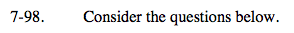$\frac{8}{1}\cdot\frac{?}{?}=\frac{1}{1}$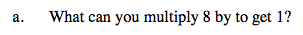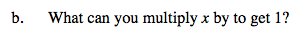See part (a).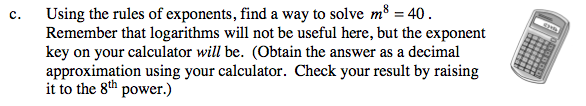Many calculators require the parentheses for this step.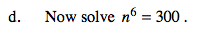Use the method you found in part (c).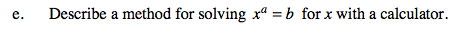Generalize the method you found in part (c).